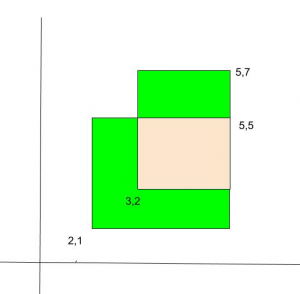Total area of two overlapping rectangles

• Difficulty Level : Medium
• Last Updated : 27 Apr, 2021

Given two overlapping rectangles on a plane. We are given bottom left and top right points of the two rectangles. We need to find the total area (Green and pink areas in the below diagram).Examples:

Input : Point l1 = {2, 2}, r1 = {5, 7};
Point l2 = {3, 4}, r2 = {6, 9};
Output :Total Area = 24

Input : Point l1 = {2, 1}, r1 = {5, 5};
Point l2 = {3, 2}, r2 = {5, 7};
Output :Total Area = 16

We basically add areas of two rectangles. This includes the intersecting part twice, so we subtract the area of intersecting part.

Total Area = (Area of 1st rectangle +
Area of 2nd rectangle) -
Area of Intersecting part

Area of Rectangle = x_distance * y_distance

Where,
x_distance for 1st rectangle = abs(l1.x – r1.x)
y_distance for 1st rectangle = abs(l1.y – r1.y)

Similarly, we can compute area of 2nd rectangle.

For area of intersecting part,
x_distance for intersecting rectangle = min(r1.x, r2.x) – max(l1.x, l2.x)
y_distance for 1st rectangle = min(r1.y, r2.y) – max(l1.y, l2.y)

If the x_distance or y_distance is negative, then the two rectangles do not intersect. In that case, overlapping area is 0.

Below is the implementation of the above approach:

C++

 // C++ program to find total area of two// overlapping Rectangles#include using namespace std; struct Point {    int x, y;}; // Returns Total Area  of two overlap// rectanglesint overlappingArea(Point l1, Point r1,                    Point l2, Point r2){    // Area of 1st Rectangle    int area1 = abs(l1.x - r1.x)      * abs(l1.y - r1.y);     // Area of 2nd Rectangle    int area2 = abs(l2.x - r2.x)      * abs(l2.y - r2.y);     // Length of intersecting part i.e    // start from max(l1.x, l2.x) of    // x-coordinate and end at min(r1.x,    // r2.x) x-coordinate by subtracting    // start from end we get required    // lengths    int x_dist = min(r1.x, r2.x)                  - max(l1.x, l2.x);    int y_dist = (min(r1.y, r2.y)                  - max(l1.y, l2.y));    int areaI = 0;    if( x_dist > 0 && y_dist > 0 )    {        areaI = x_dist * y_dist;    }         return (area1 + area2 - areaI);} // Driver Codeint main(){    Point l1 = { 2, 2 }, r1 = { 5, 7 };    Point l2 = { 3, 4 }, r2 = { 6, 9 };     // Function Call    cout << overlappingArea(l1, r1, l2, r2);    return 0;}

Java

 // Java program to find total area of two// overlapping Rectanglesclass GFG {     static class Point {        int x, y;         public Point(int x, int y)        {            this.x = x;            this.y = y;        }    };     // Returns Total Area of two overlap    // rectangles    static int overlappingArea(Point l1, Point r1,                               Point l2, Point r2)    {        // Area of 1st Rectangle        int area1            = Math.abs(l1.x - r1.x)              * Math.abs(l1.y - r1.y);         // Area of 2nd Rectangle        int area2            = Math.abs(l2.x - r2.x)              * Math.abs(l2.y - r2.y);         // Length of intersecting part i.e        // start from max(l1.x, l2.x) of        // x-coordinate and end at min(r1.x,        // r2.x) x-coordinate by subtracting        // start from end we get required        // lengths         int x_dist = (Math.min(r1.x, r2.x)                      - Math.max(l1.x, l2.x);        int y_dist = (Math.min(r1.y, r2.y)                 - Math.max(l1.y, l2.y);        int areaI = 0;        if( x_dist > 0 && y_dist > 0 )        {            areaI = x_dist * y_dist;        }         return (area1 + area2 - areaI);    }     // Driver Code    public static void main(String[] args)    {        Point l1 = new Point(2, 2), r1 = new Point(5, 7);        Point l2 = new Point(3, 4), r2 = new Point(6, 9);         // Function Call        System.out.println(overlappingArea(l1, r1, l2, r2));    }} // This code is contributed by PrinciRaj1992

Python3

 # Python program to find total area of two# overlapping Rectangles# Returns Total Area  of two overlap#  rectangles  def overlappingArea(l1, r1, l2, r2):    x = 0    y = 1     # Area of 1st Rectangle    area1 = abs(l1[x] - r1[x]) * abs(l1[y] - r1[y])     # Area of 2nd Rectangle    area2 = abs(l2[x] - r2[x]) * abs(l2[y] - r2[y])     ''' Length of intersecting part i.e         start from max(l1[x], l2[x]) of         x-coordinate and end at min(r1[x],        r2[x]) x-coordinate by subtracting         start from end we get required         lengths '''    x_dist = (min(r1[x], r2[x]) -              max(l1[x], l2[x]))     y_dist = (min(r1[y], r2[y]) -              max(l1[y], l2[y]))    areaI = 0    if x_dist > 0 and y_dist > 0:        areaI = x_dist * y_dist     return (area1 + area2 - areaI)  # Driver's Codel1 = [2, 2]r1 = [5, 7]l2 = [3, 4]r2 = [6, 9] # Function callprint(overlappingArea(l1, r1, l2, r2)) # This code is contributed by Manisha_Ediga

C#

 // C# program to find total area of two// overlapping Rectanglesusing System; class GFG {    public class Point {        public int x, y;         public Point(int x, int y)        {            this.x = x;            this.y = y;        }    };     // Returns Total Area of two overlap    // rectangles    static int overlappingArea(Point l1, Point r1, Point l2,                               Point r2)    {        // Area of 1st Rectangle        int area1            = Math.Abs(l1.x - r1.x) * Math.Abs(l1.y - r1.y);         // Area of 2nd Rectangle        int area2            = Math.Abs(l2.x - r2.x) * Math.Abs(l2.y - r2.y);         // Length of intersecting part i.e        // start from max(l1.x, l2.x) of        // x-coordinate and end at min(r1.x,        // r2.x) x-coordinate by subtracting        // start from end we get required        // lengths        int x_dist            = (Math.Min(r1.x, r2.x) - Math.Max(l1.x, l2.x));        int y_dist            = (Math.Min(r1.y, r2.y) - Math.Max(l1.y, l2.y));        int areaI = 0;        if (x_dist > 0 && y_dist > 0) {            areaI = x_dist * y_dist;        }         return (area1 + area2 - areaI);    }     // Driver Code    public static void Main(String[] args)    {        Point l1 = new Point(2, 2), r1 = new Point(5, 7);        Point l2 = new Point(3, 4), r2 = new Point(6, 9);         // Function Call        Console.WriteLine(overlappingArea(l1, r1, l2, r2));    }} // This code is contributed by PrinciRaj1992

Javascript


Output
24

My Personal Notes arrow_drop_up